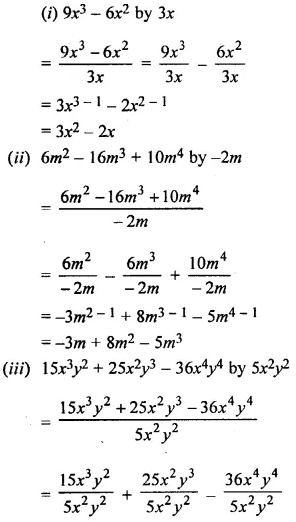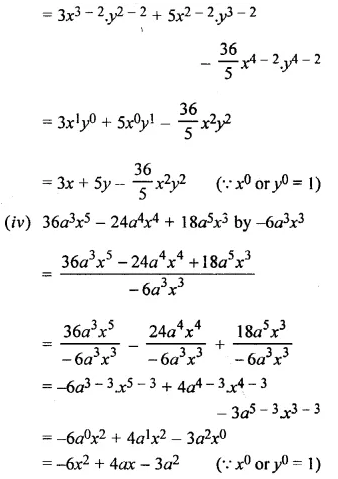# Selina Concise Mathematics Class 6 ICSE Solutions Chapter 19 Fundamental Operations

## Selina Concise Mathematics Class 6 ICSE Solutions Chapter 19 Fundamental Operations (Related to Algebraic Expressions)

Selina Publishers Concise Mathematics Class 6 ICSE Solutions Chapter 19 Fundamental Operations (Related to Algebraic Expressions)

### Fundamental Operations Exercise 19A – Selina Concise Mathematics Class 6 ICSE Solutions

Question 1.
Fill in the blanks :
(i) 5 + 4 = ………… and 5x + 4x = ………….
(ii) 12 + 18 = ………… and 12x2y + 18x2y = ………….
(iii) 7 + 16 = ………….. and 7a + 16b = …………
(iv) 1 + 3 = ………… and x2y + 3xy2 = ………..
(v) 7 – 4 = …………… and 7ab – 4ab = …………..
(vi) 12 – 5 = ………… and 12x – 5y = ……………
(vii) 35 – 16 = ………….. and 35ab – 16ba = ………….
(viii) 28 – 13 = …………. and 28ax2 – 13a2x = ………….
Solution: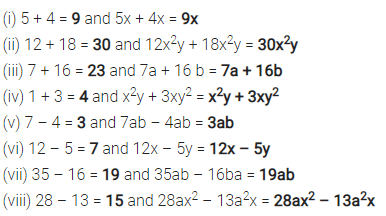Question 2.
Fill in the blanks :
(i) The sum of – 2 and – 5 = …………. and the sum of – 2x and – 5x = …………….
(ii) The sum of 8 and – 3 = ………….. and the sum of 8ab and – 3ab = ………….
(iii) The sum of – 15 and – 4 = …………….. and the sum of – 15x and -4y = ………………
(iv) 15 + 8 + 3 = ……….. and 15x + 8y + 3x = …………….
(v) 12 – 9 + 15 = …………… and 12ab – 9ab + 15ba = ……………..
(vi) 25 – 7 – 9 = and 25xy – 7xy – 9yx = ……………
(vii) – 4 – 6 – 5 = …………. and – 4ax – 6ax – 5ay = …………….
Solution:Question 3.
(i) 8xy and 3xy
(ii) 2xyz, xyz and 6xyz
(iii) 2a, 3a and 4b
(iv) 3x and 2y
(v) 5m, 3n and 4p
(vi) 6a, 3a and 9ab
(vii) 3p, 4q and 9q
(viii) 5ab, 4ba and 6b
(ix) 50pq, 30pq and 10pr
(x) – 2y, – y and – 3y
(xi) – 3b and – b
(xii) 5b, – 4b and – 10b
(xiii) – 2c, – c and – 5c
Solution: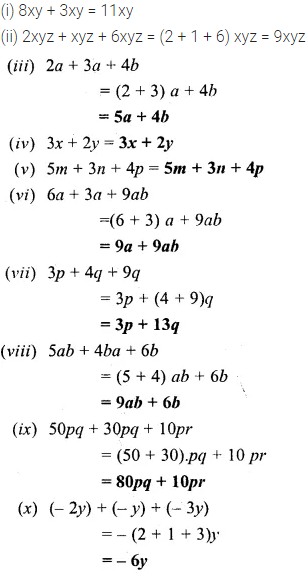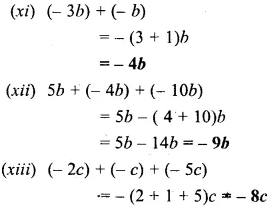Question 4.
Evaluate :Solution: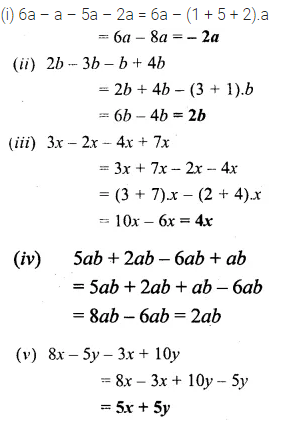Question 5.
Evaluate :Solution: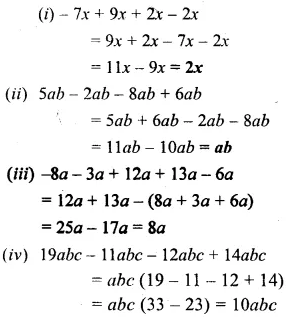Question 6.
Subtract the first term from the second :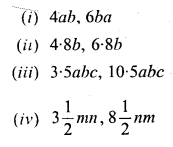Solution: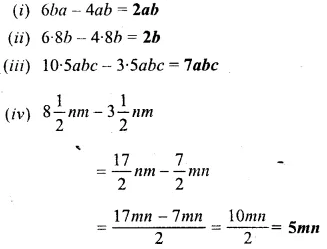Question 7.
Simplify :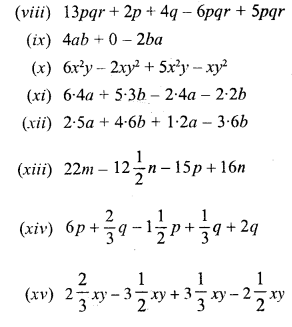Solution: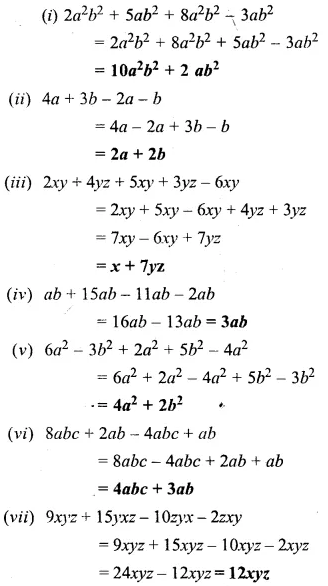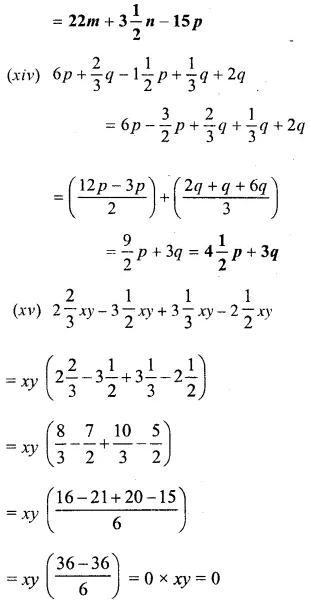### Fundamental Operations Exercise 19B – Selina Concise Mathematics Class 6 ICSE Solutions

Question 1.
Find the sum of :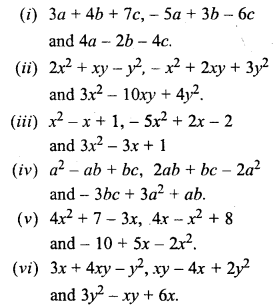Solution: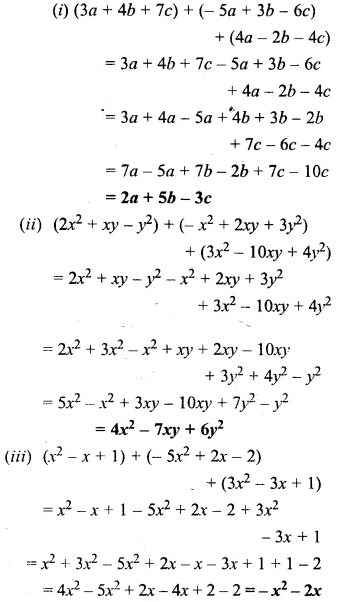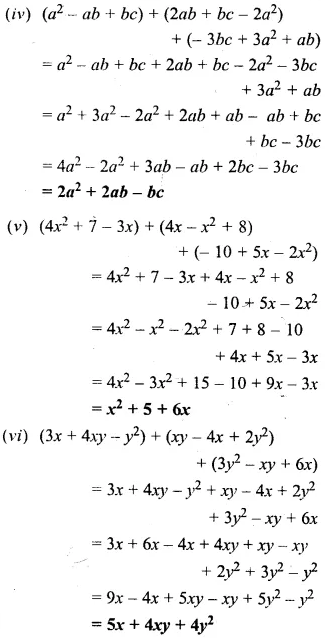Question 2.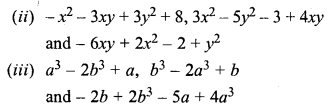Solution:Question 3.
Evaluate :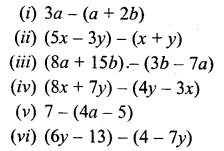Solution: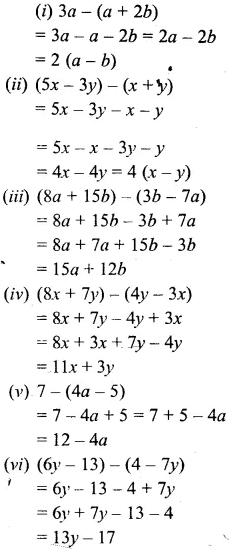Question 4.
Subtract :Solution: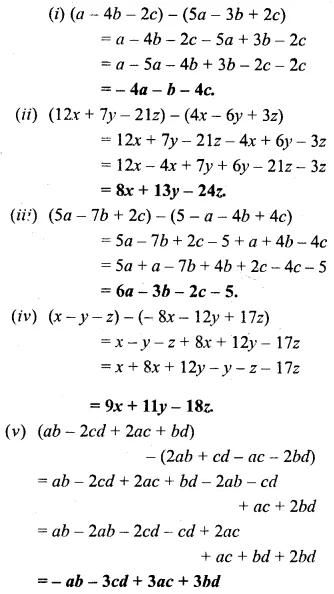Question 5.Solution: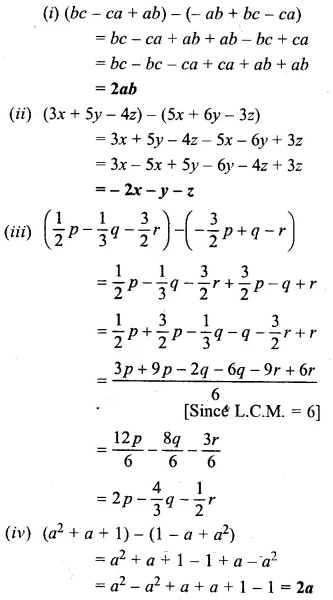Question 6.
From the sum of x + y – 2z and 2x – y + z subtract x + y + z.
Solution: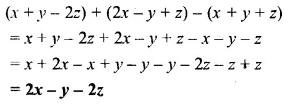Question 7.
From the sum of 3a – 2b + 4c and 3b – 2c subtract a – b – c.
Solution: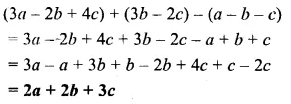Question 8.
Subtract x – 2y – z from the sum of 3x – y + z and x + y – 3z.
Solution:Question 9.
Subtract the sum of x + y and x – z from the sum of x – 2z and x + y + z
Solution: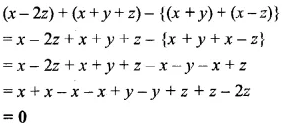Question 10.
By how much should x + 2y – 3z be increased to get 3x ?
Solution:Question 11.
The sum of two expressions is 5x2 – 3y2. If one of them is 3x2 + 4xy – y2, find the other.
Solution: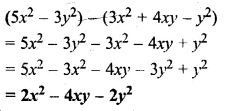Question 12.
The sum of two expressions is 3a2 + 2ab – b2. If one of them is 2a2 + 3b2, find the other.
Solution: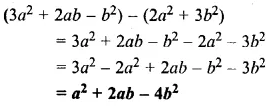### Fundamental Operations Exercise 19C – Selina Concise Mathematics Class 6 ICSE Solutions

Question 1.
Fill in the blanks :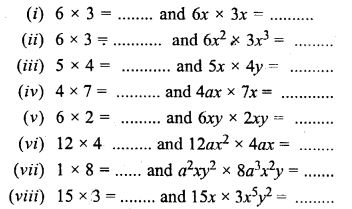Solution:Question 2.
Fill in the blanks :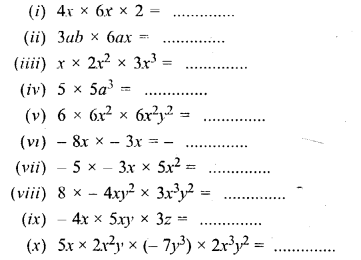Solution:Question 3.
Find the value of :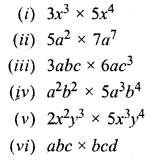Solution: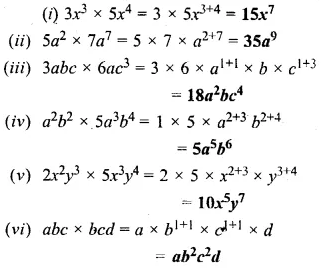Question 4.
Multiply :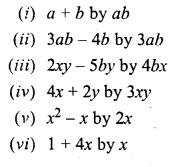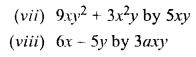Solution: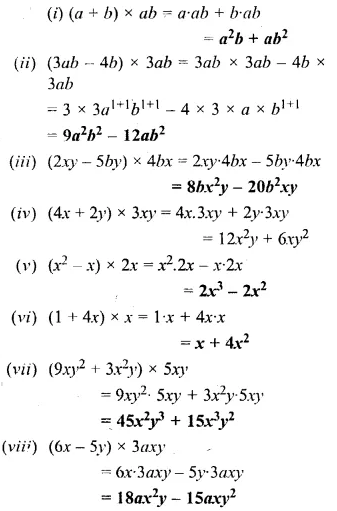Question 5.
Multiply :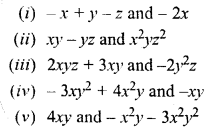Solution: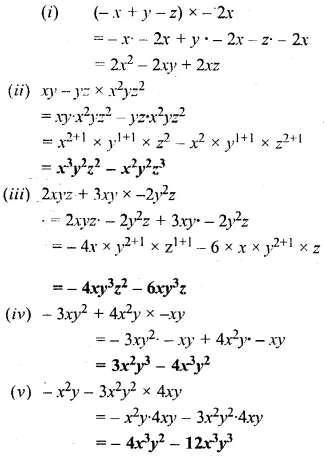Question 6.
Multiply :Solution: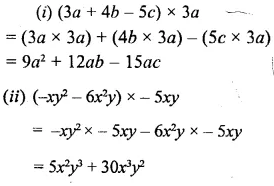Question 7.
Multiply :
(i) x + 2 and x + 10
(ii) x + 5 and x – 3
(iii) x – 5 and x + 3
(iv) x – 5 and x – 3
(v) 2x+ y and x+ 3y
(vi) (3x – 5y) and (x + 6y)
(vii) (x + 9y) and (x – 5y)
(viii) (2x + 5y) and (2x + 5y)
Solution: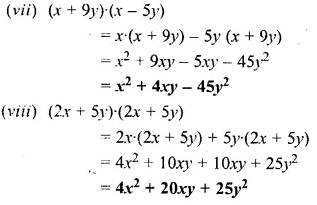Question 8.
Multiply :Solution:Question 9.
Find the product of :Solution: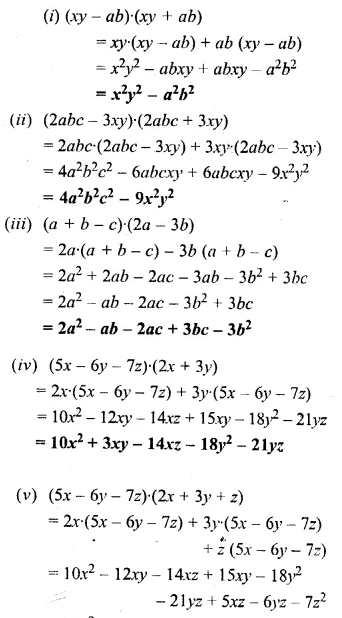### Fundamental Operations Exercise 19D – Selina Concise Mathematics Class 6 ICSE Solutions

Question 1.
Divide :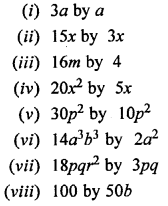Solution: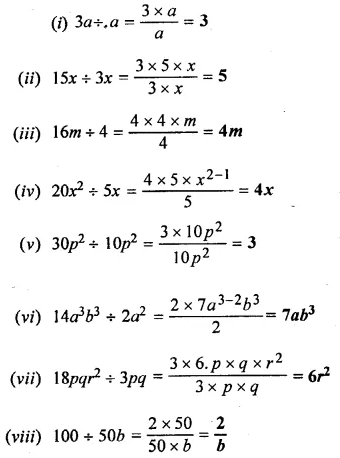Question 2.
Simplify :Solution: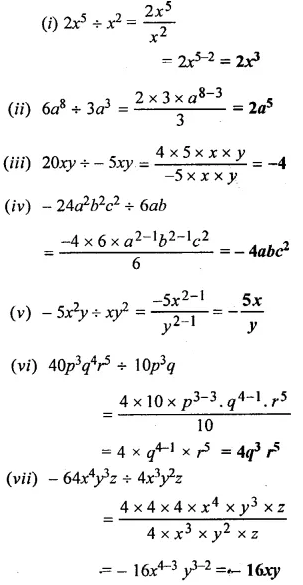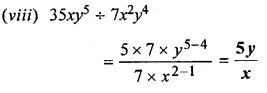Question 3.
Divide :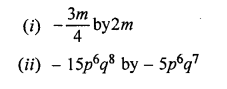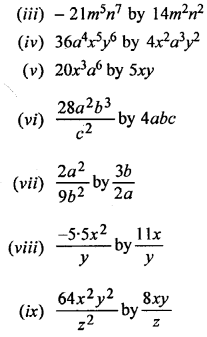Solution:Question 4.
Simplify :Solution: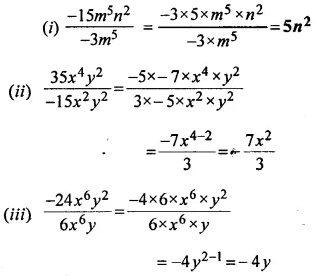Question 5.
Divide :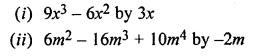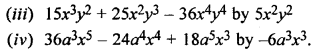Solution: